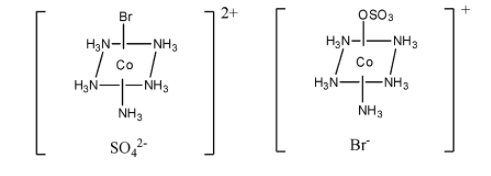What is ionization isomerism? Give one example.Verified
145.8k+ views
Hint: Isomerism is defined as the process where more than just one compound contains the same chemical formulas, but they have different chemical structures. There exists two types of isomerism namely structural isomerism and stereoisomerism.

Complete step-by-step solution:
Let us see the difference between structural and stereoisomers:
> The isomers in which the molecules having the same molecular formula are arranged with different bonding patterns and atomic orientation are called the structural isomerism. Structural isomerism is classified as chain isomers, positional isomers, functional isomers, metamerism, tautomerism and ring chain.
> The isomers in which the molecular bonds are placed in the same order and where the spatial arrangements differ are called the stereoisomers. Stereoisomers are classified as geometrical isomers and optical isomers.
> Ionisation isomerism is shown by such compounds in solution that give different ions although they possess the same chemical composition. Ionisation isomerism is seen to occur in a complex salt when its counter ion is a potential ligand and this counter ion can displace a ligand making it to behave or become a counter ion.
> One example of ionisation isomerism is $\left[ {{\rm{Co}}{{\left( {{\rm{N}}{{\rm{H}}_{\rm{3}}}} \right)}_{\rm{5}}}{\rm{S}}{{\rm{O}}_{\rm{4}}}} \right]{\rm{Br}}$ and $\left[ {{\rm{Co}}{{\left( {{\rm{N}}{{\rm{H}}_{\rm{3}}}} \right)}_{\rm{5}}}{\rm{Br}}} \right]{\rm{S}}{{\rm{O}}_{\rm{4}}}$.

> The structure of both the isomers $\left[ {{\rm{Co}}{{\left( {{\rm{N}}{{\rm{H}}_{\rm{3}}}} \right)}_{\rm{5}}}{\rm{S}}{{\rm{O}}_{\rm{4}}}} \right]{\rm{Br}}$ and $\left[ {{\rm{Co}}{{\left( {{\rm{N}}{{\rm{H}}_{\rm{3}}}} \right)}_{\rm{5}}}{\rm{Br}}} \right]{\rm{S}}{{\rm{O}}_{\rm{4}}}$are given below.$\left[ {{\rm{Co}}{{\left( {{\rm{N}}{{\rm{H}}_{\rm{3}}}} \right)}_{\rm{5}}}{\rm{Br}}} \right]{\rm{S}}{{\rm{O}}_{\rm{4}}}$ $\,\,\,\,\,\,\,\,\,\,\,\,\,\,\,\,\,\,\,\,\,\,\,\,\,\,\,\,\,\,\,\,\,\,\,\,\,\,\,\,\,\,\,$ $\left[ {{\rm{Co}}{{\left( {{\rm{N}}{{\rm{H}}_{\rm{3}}}} \right)}_{\rm{5}}}{\rm{S}}{{\rm{O}}_{\rm{4}}}} \right]{\rm{Br}}$

We can prepare these ionisation isomers in the following way:
$\begin{array}{l}\left[ {{\rm{CoBr}}{{\left( {{\rm{N}}{{\rm{H}}_{\rm{3}}}} \right)}_{\rm{5}}}} \right]{\rm{S}}{{\rm{O}}_{\rm{4}}} \to {\left[ {{\rm{CoBr}}{{\left( {{\rm{N}}{{\rm{H}}_{\rm{3}}}} \right)}_{\rm{5}}}} \right]^{2 + }} + {\rm{SO}}_4^{2 - }\\{\rm{ Red - Violet}}\end{array}$
$\begin{array}{l}\left[ {{\rm{Co S}}{{\rm{O}}_{\rm{4}}}{{\left( {{\rm{N}}{{\rm{H}}_{\rm{3}}}} \right)}_{\rm{5}}}} \right]{\rm{Br}} \to {\left[ {{\rm{Co SO}}_4^{2 - }{{\left( {{\rm{N}}{{\rm{H}}_{\rm{3}}}} \right)}_{\rm{5}}}} \right]^ + } + {\rm{B}}{{\rm{r}}^ - }\\{\rm{ Red}}\end{array}$

Note: Students may get confused about how ionisation isomerism looks like. In ionisation isomerism, the compounds in solution give different ions although they possess the same chemical composition. The difference in ionisation isomerism is due to the difference in position of placing the atoms or groups in the coordination sphere.